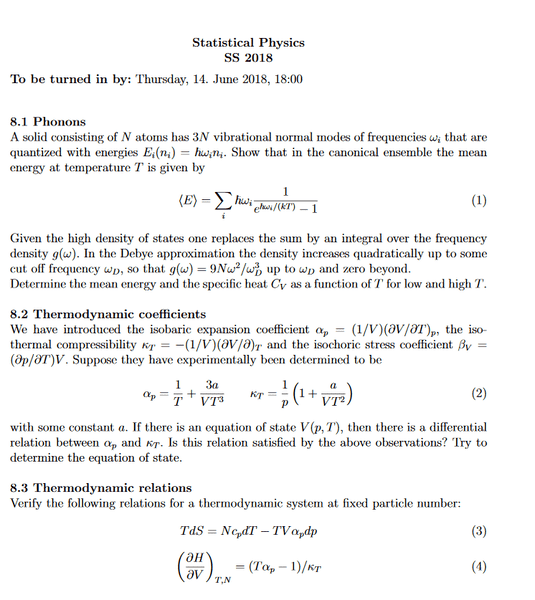# Determing equation of state from thermodynamic coefficients

## Homework Statement

The isobaric expansion coefficient and the isothermal compressibility are given by:
$$\alpha_p = (1/V)(\partial V/\partial T)_p \quad \kappa_T = -(1/V)(\partial V / \partial p)_T$$
Suppose they have experimentally been determined to be: $$\alpha_p = \frac{1}{T} + \frac{3a}{VT^3} \quad \kappa_T = \frac{1}{p}\left(1+\frac{a}{VT^2}\right)$$ with some constant a. Try to determine the equation of state.

## Homework Equations

$$dV(T,p) = \frac{\partial V}{\partial T}dT + \frac{\partial V}{\partial p}dp$$
$$\frac{\partial^2 F}{\partial V^2} = \frac{1}{\kappa_T V}$$ (Not sure if the last one is useful)

## The Attempt at a Solution

I calculated the free energy by plugging in ##\kappa_T## and then solving the equation for ##F## giving: ##F(V,p,T) = pV ln(a+T^2V)+\left(\frac{ap}{T^2}\right)ln(a+T^2V)+c_2V+c_1##
Using the first two equations for ##\alpha_p## and ##\kappa_T## I got $$V(T,p)=\frac{-a~ln~p}{(1+ln~p)T^2}+c,\quad V(T)=\frac{-3a}{2T^2(1-ln~T)}+c$$ by integrating over ##T## for ##\alpha_p## and over ##T## for ##\kappa_T##.

But this approach doesn't seem to make much sense since the two equations for V differ.

I'm a bit curious about the source of this problem. Could you share the source, please?

I'm a bit curious about the source of this problem. Could you share the source, please?

I don't have the source but I attached a screenshot of the problem sheet as it was handed out.#### Attachments

Thank you. I'm a bit confused about how your calculation of F leads to the expressions you wrote for V. But the first relevant equation you wrote is very useful because you can replace ∂V/∂P by -VκT and replace ∂V/∂T by Vα. Now the question in the problem sheet asks about something first before trying to evaluate V(T,P) which is whether these experimentally determined expressions make sense. One needs to recall a condition on the second derivatives of V(T,P) (or any thermodynamic state function)

The second part of the question, evaluating V(T,P) should straightforward integration of the partial differential expression you wrote:

$$dV(T,p) = \frac{\partial V}{\partial T}dT + \frac{\partial V}{\partial p}dp$$

Thank you. I'm a bit confused about how your calculation of F leads to the expressions you wrote for V. But the first relevant equation you wrote is very useful because you can replace ∂V/∂P by -VκT and replace ∂V/∂T by Vα. Now the question in the problem sheet asks about something first before trying to evaluate V(T,P) which is whether these experimentally determined expressions make sense. One needs to recall a condition on the second derivatives of V(T,P) (or any thermodynamic state function)

The second part of the question, evaluating V(T,P) should straightforward integration of the partial differential expression you wrote:

My calculation for ##F## didn't really seem to go anywhere, added it to the question just in case it could have been useful.

##dV(T,p) = \frac{\partial V}{\partial T}dT + \frac{\partial V}{\partial p}dp \implies \int \frac{dv}{v} = -\int \kappa_T dp + \int \alpha_p dT \implies## $$ln~V = -\left(1+\frac{a}{VT^2}\right)ln~p + ln~T-\frac{3a}{2VT^2}+c$$ but I can't seem to solve this for V, does this mean that they really don't make sense as you said?

You cannot integrate this way, in the presence of V(T,P) in the right hand side. You need to have T,P only in the right hand side.

To check the validity of the expressions you should invoke the symmetry of the mixed second derivatives. Again when you calculate the second derivative make sure that you treat V as a function of T,P.

You cannot integrate this way, in the presence of V(T,P) in the right hand side. You need to have T,P only in the right hand side.

To check the validity of the expressions you should invoke the symmetry of the mixed second derivatives. Again when you calculate the second derivative make sure that you treat V as a function of T,P.

I cannot seem to get the differential fiddled into parts like: ##f(V)dV = g(T)dT + h(p)dp## the best I could do is something like: $$dV+V\left(\frac{dp}{p}-\frac{dT}{T}\right) = \frac{-adp}{T^2}+\frac{3adT}{T^3}$$ or $$\frac{1}{V}\left(dV+\frac{adp}{T^2}-\frac{3adT}{T^3}\right)= \frac{-dp}{p}+\frac{dT}{T}$$

For the second mixed partial derivatives I got: $$\frac{\partial^2 V}{\partial p \partial T} = \frac{\partial \alpha_p V}{\partial p} = 0 + \alpha_p\frac{\partial V}{\partial p} = -\alpha_p V\kappa_T$$ and $$\frac{\partial^2 V}{\partial T \partial p} = -\frac{\partial V \kappa_T}{\partial T} = -V\alpha_p\kappa_T + \frac{2a}{pT^3}$$

which means they differ by ##\frac{2a}{pT^3}##.
In the lecture we just always assume they are equal, e.g the order doesn't matter. But does this mean then that there is no equation of state?

If there is no value for the constant a that ensures the equality of the two mixed derivative, then it looks to me that there is inconsistency in the experimental data. Note that a = 0, leads to the equation of state of the ideal gas as you can check by integration of dV(T,P).

•AwesomeTrains